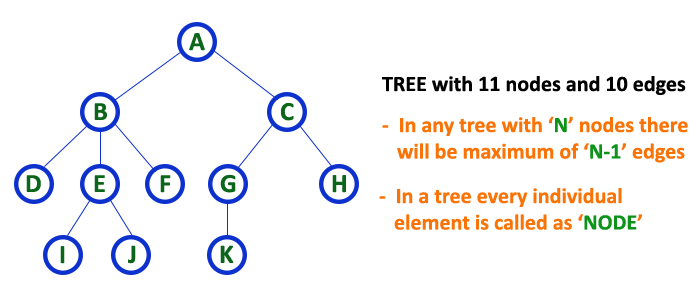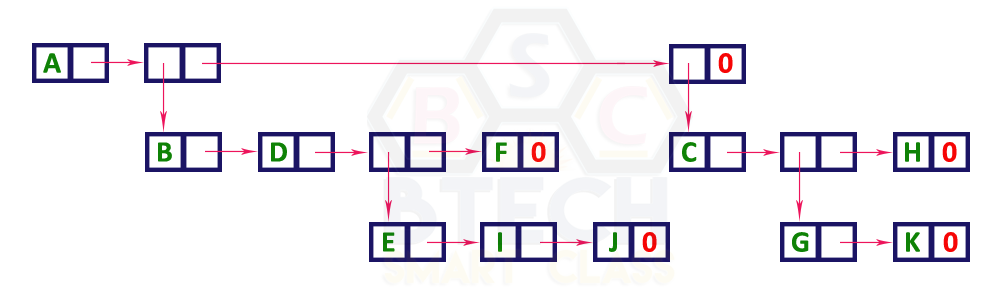The perfect place for easy learning...

×

# Tree Representations

A tree data structure can be represented in two methods. Those methods are as follows...

1. List Representation
2. Left Child - Right Sibling Representation

Consider the following tree...# 1. List Representation

In this representation, we use two types of nodes one for representing the node with data called 'data node' and another for representing only references called 'reference node'. We start with a 'data node' from the root node in the tree. Then it is linked to an internal node through a 'reference node' which is further linked to any other node directly. This process repeats for all the nodes in the tree.

The above example tree can be represented using List representation as follows...# 2. Left Child - Right Sibling Representation

In this representation, we use a list with one type of node which consists of three fields namely Data field, Left child reference field and Right sibling reference field. Data field stores the actual value of a node, left reference field stores the address of the left child and right reference field stores the address of the right sibling node. Graphical representation of that node is as follows...In this representation, every node's data field stores the actual value of that node. If that node has left a child, then left reference field stores the address of that left child node otherwise stores NULL. If that node has the right sibling, then right reference field stores the address of right sibling node otherwise stores NULL.
The above example tree can be represented using Left Child - Right Sibling representation as follows...